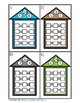# Multiplication and Division Fact Family HousesSubject
Resource Type
File Type

PDF

(2 MB|19 pages)
Standards
• Product Description
• StandardsNEW
Multiplication and Division Fact Family Houses include facts to 12. Students write the fact They are in color and black and white. The black and white ones can be printed on colored cardstock to save you ink.

These fact families are perfect for centers, rotations, or stations, early finishers, small groups, or print a page and give each student a page to complete in whole group. Then it can be used as an assessment.

Answer keys and recording sheets are included. Students will have to write small to fit all 4 facts on each line or have them write on lined paper, which may be easier.

Other Products You May Enjoy:

Multiplication Wheels
Beach Division Race

Thanks for hoppin' by,

Brian Hopkins
Hopkins' Hoppin' Happenings
Apply properties of operations as strategies to multiply and divide. Examples: If 6 × 4 = 24 is known, then 4 × 6 = 24 is also known. (Commutative property of multiplication.) 3 × 5 × 2 can be found by 3 × 5 = 15, then 15 × 2 = 30, or by 5 × 2 = 10, then 3 × 10 = 30. (Associative property of multiplication.) Knowing that 8 × 5 = 40 and 8 × 2 = 16, one can find 8 × 7 as 8 × (5 + 2) = (8 × 5) + (8 × 2) = 40 + 16 = 56. (Distributive property.)
Determine the unknown whole number in a multiplication or division equation relating three whole numbers. For example, determine the unknown number that makes the equation true in each of the equations 8 × ? = 48, 5 = __ ÷ 3, 6 × 6 = ?.
Total Pages
19 pages
Included
Teaching Duration
N/A
Report this Resource to TpT
Reported resources will be reviewed by our team. Report this resource to let us know if this resource violates TpT’s content guidelines.